Mathematics - All About Trigonometry (Part 2)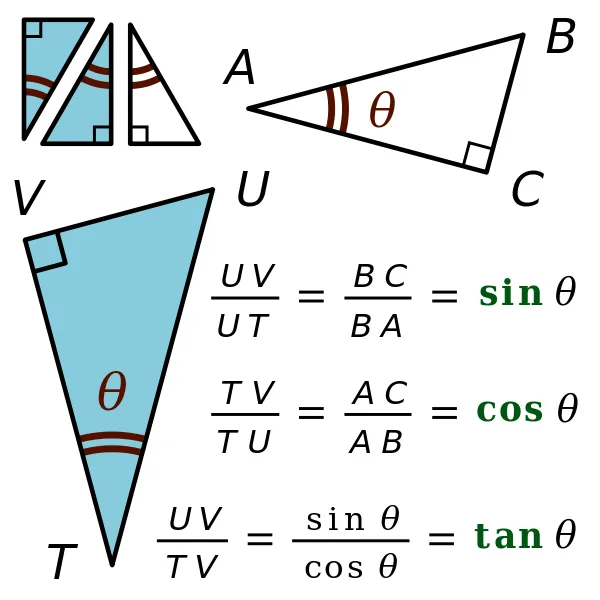[Image1]

Introduction

Hey it's a me again @drifter1!

Today we continue with the small series about Trigonometry. I suggest reading the first article before getting into this one. Today we will cover Trigonometric Functions and how Right-Angled Triangles can be solved using them...

So, without further ado, let's dive straight into it!

Trigonometric Functions

Based on a Right-Angled Triangle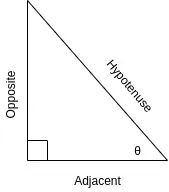[Custom Figure using draw.io]

Having a right-angled triangle in mind, for any angle θ the three main trigonometric functions are defined as "soh-cah-toa":The size of the sides doesn't matter, as only the angle changes the ratio.

Similarly, we can also define the less-used cosecant (csc), secant (sec) and cotangent (cot):Unit Circle

A unit circle is a circle with radius 1 and center at O (0, 0) in the Cartesian Space:[Custom Figure using GeoGebra]

Because the radius is 1, we can directly measure the sine, cosine and tangent, as well as all the other trigonometric functions.

Pythagoras's Theorem

An article about Trigonometry wouldn't be complete without Pythagoras's Theorem:

For a right-angled triangle, the square of the long side (hypotenuse) equals the sum of the squares of the other two sides.

In the case of the unit circle: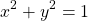And since x = cos θ and y = sin θ, we easily derive the following useful identity:which is true for any angle θ.

30°, 45° and 60° Angles

The values of the sine, cosine and tangent of the 30°, 45° and 60° can be easily remembered:

• sinus goes √1, √2, √3, divided by 2
• cosinus goes √3, √2, √1 divided by 2
• tangent is the division of sinus and cosinus (tan = sin/cos)
In tabular form:In the two-dimensional Cartesian Coordinate System we define the position of a point on a graph by how far along (x) and how far up (y) it is in respect to the center O (0, 0).

Including negative values for x and y, we easily divide this space into 4 pieces called Quadrants (counter-clockwise direction):

• Quadrant I - both x and y are positive
• Quadrant II - x is negative and y is positive
• Quadrant III - both x and y are negative
• Quadrant IV - x is positive and y is negative
Visually:[Custom Figure using GeoGebra]
• A (3, 9) is in Quadrant I
• B (-7, 6) is in Quadrant II
• C (-4, -5) is in Quadrant III
• D (8, -2) is in Quadrant IV

Sinus, Cosine and Tangent in the Four Quadrants

• Quadrant I - all trigonometric functions are positive
• Quadrant II - only sinus positive
• Quadrant III - only tangent positive
• Quadrant IV - only cosinus positive
So, there is a pattern: "A-S-T-C", that is easy to remember.

Angles with same Trigonometric Function Result

Trigonometric Functions are periodic functions, meaning that two different angles return the same result for sin, cos and tan. Simple relations to remember are:Inverse Trigonometric Functions

Let's now get into inverse trigonometric functions. In the inverse function we insert the value of the trigonometric function and it returns the angle θ.

Thus, we define the inverse sine, inverse cosine and inverse tangent as:Infinite Solutions

Of course there are infinite answers, two per 360° degree circle to be exact, and repeating after that.

Mathematically the solution of x = sinθ or θ = arctan(x) can be written:Similarly, the solution of x = cosθ or θ = arccos(x) is:And finally the solution of x = tanθ or θ = arctan(x) is: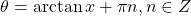Solving Right Triangles

The simplest triangles to solve are those with a right angle.

There are two types of unknown values:

1. Unknown Angles
2. Unknown Sides

Finding an unknown angle

To calculate an unknown angle in a right-angled triangle we have to know the lengths of at least two of its sides.

• If all sides are known then we can use any of the trigonometric functions (sin, cos, tan)
• If only two are known then we have to identify which of the trigonometric functions has to be used:
• Opposite and Hypotenuse known → Sinus
• Adjacent and Hypotenuse known → Cosinus
• Adjacent and Opposite known → Tangent

Finding an unknown side

To find an unknown side in a right-angled triangle we have to know:

• the length of at least one side
• one angle (not including the right-angle!)
If two sides are known then we can directly apply Pythagoras's Theroem to calculate the remaining side!

If not, then we of course have to choose the correct trigonometric function to calculate the side that we want to find. The choice depends on which angle we know and the relation of the known side to that angle.

For example if the side that we know is opposite to the known angle, and the side that we want to find is the Hypotenuse, then we have to use the Sinus Function.

Full-on Examples

One side and one angle known

Let's consider the following Triangle: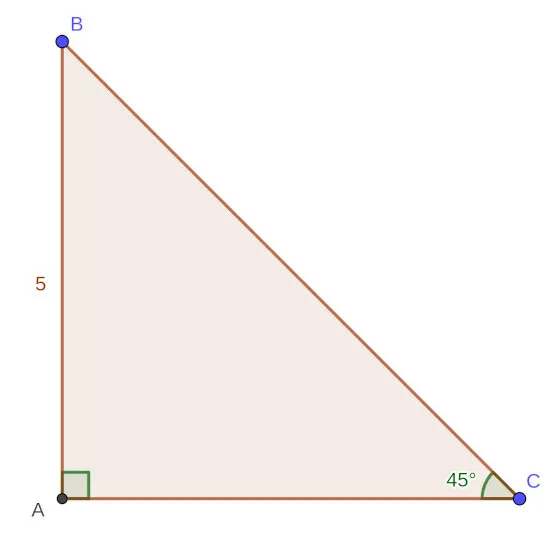[Custom Figure using GeoGebra]

We only know one angle (excluding the right-angle) and one side. Let's find all the remaining angles and sides!

Let's start with the Hypotenuse. We know the opposite side of the angle of 45° and so to calculate the Hypotenuse we have to use the Sinus function:Using Pythagoras's Theorem we can now calculate the adjacent side AC:And because the angles sum up to 180° in all triangles we can also calculate the remaining angle: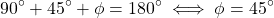Two sides known

Let's consider the following Triangle:
[Custom Figure using GeoGebra]

We only know the lengths of two sides. Let's find the remaining side and all the angles!

Using Pythagoras's Theroem we can easily calculate the Hypotenuse BC: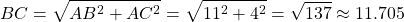The angles can be calculated using inverse trigonometric functions. Let's first calculate the bottom-left angle and then use the sum to 180° to find the upper angle.

Using the inverse tangent (arctan) we calculate (using calculator) the bottom-left angle to be:Therefore the upper angle is: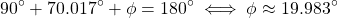RESOURCES:

Images

1.Final words | Next up

And this is actually it for today's post!

Next time we will cover how we solve any Triangle (non-right-angled), as well as Trigonometric identities, equations etc.

Also, currently my ideas for "All About" articles include:
• Geometry
• Polynomial Arithmetic
• Exponentials and Logarithms
• Rational Expressions
Basically High-School Math Refreshers for Students in Universities or maybe even Middle-Age people that have problems helping their kids out in Math at school!

See ya!Keep on drifting!
H2
H3
H4
3 columns
2 columns
1 column
Join the conversation now